# Multiplying 2-Digit Numbers by Multiples of 10

Times up!
Times up! You may finish answering the current question and then proceed to your score!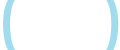Sorry, you have used all of your available hints for this lesson.
Practice Limit Reached

You've reached your daily practice limit of 12 questions.

Award InformationAwarded toQuestion HintChoose Family Member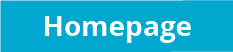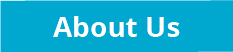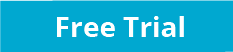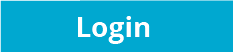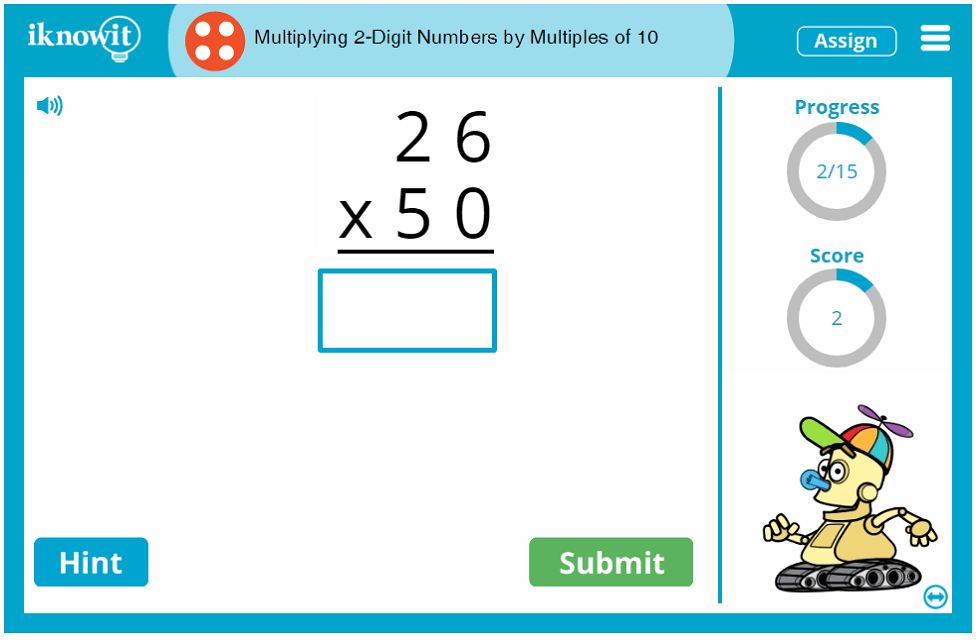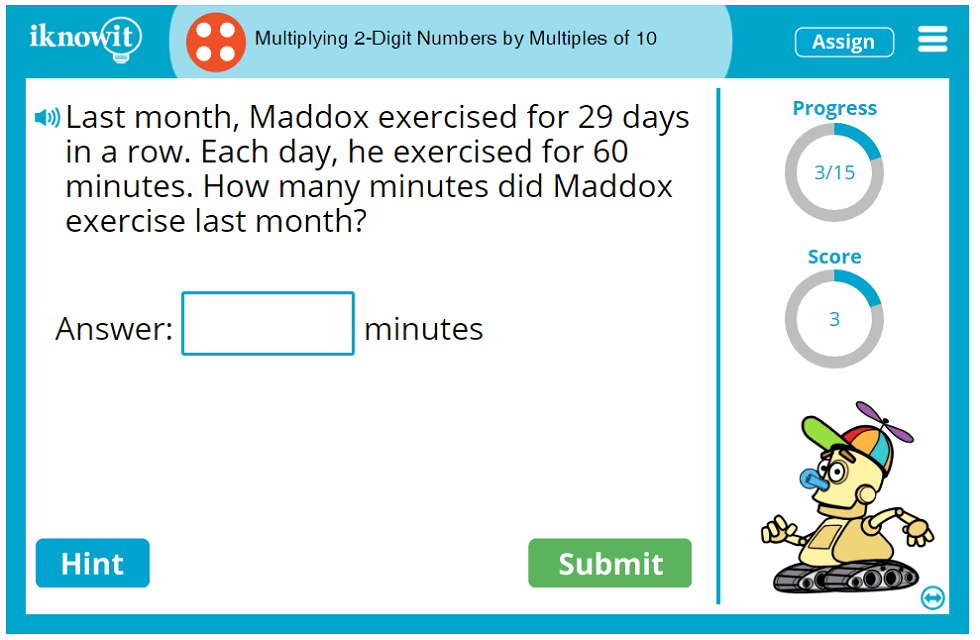## Interactive Math Lesson - Multiplying 2 Digit Numbers by Multiples of 10

In this interactive math lesson, children will practice multiplying 2-digit numbers by multiples of ten. Math problems are presented in a variety of formats in this fourth grade math activity, including vertical multiplication problems, horizontal multiplication problems, and multiplication word problems. After going through this online math game, students will gain a greater understanding of basic multiplication skills.

Here are a few examples of math problems students may encounter in this lesson: "51 x 60 =", "Multiply 46 and 30," and "Luca was baking cookies at Victoria's bakery. Each tray holds 12 cookies. Luca baked 70 trays of cookies. How many cookies did he bake?"

If students have trouble answering a math problem, they can click on the "Hint" button for a little extra help. They will be shown a relevant clue; in this case, the first step in solving the multiplication problem. If students answer a math problem incorrectly, a detailed explanation page will pop up, showing them how to solve the problem step by step, so they can learn from their mistakes along the way.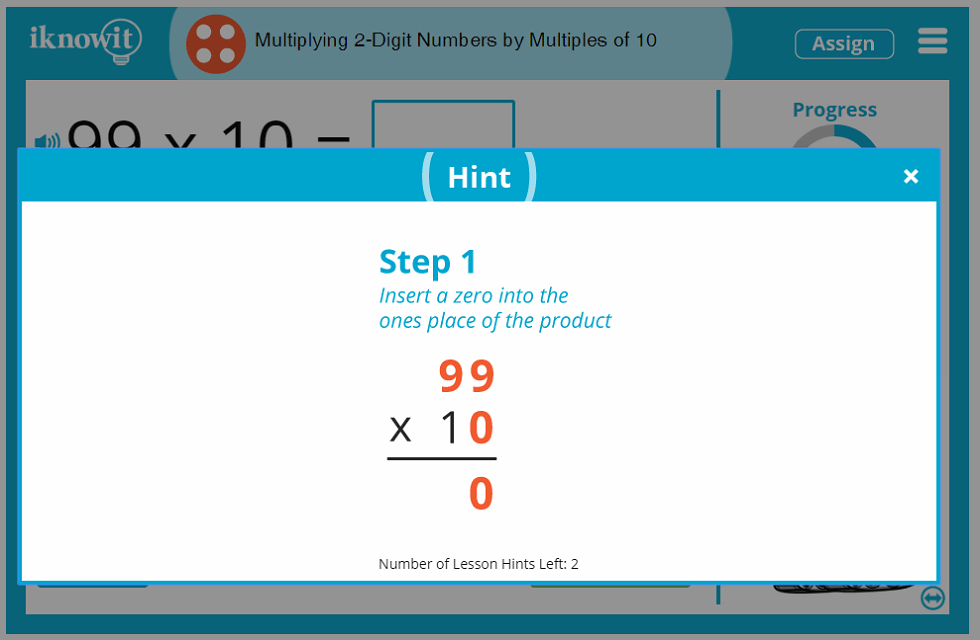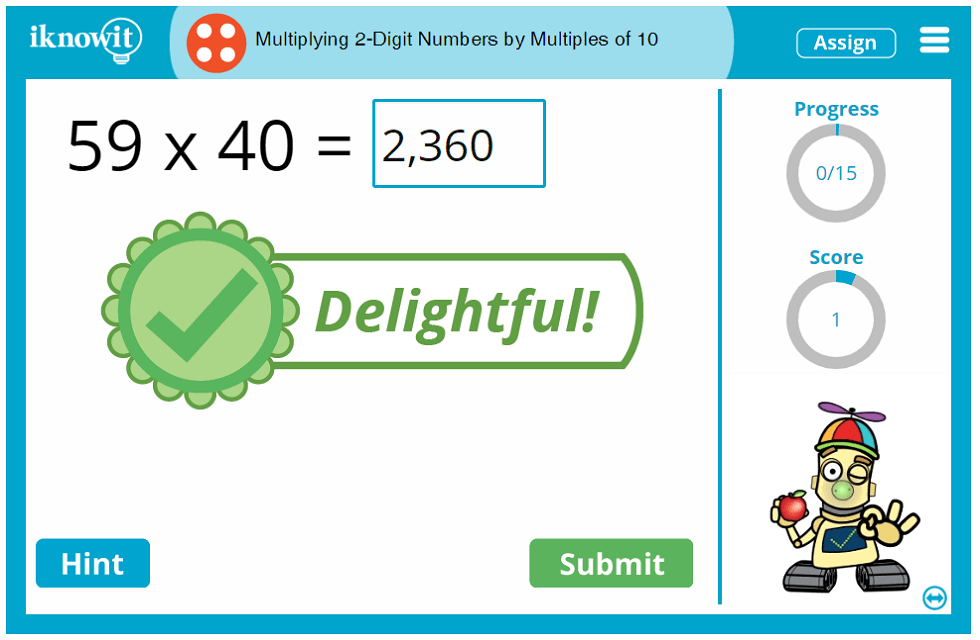## Helpful Features in 'I Know It' Math Lessons

In addition to optional hints and detailed explanations, every “I Know It” lesson offers several features to help children make the most out of their math practice time. A progress-tracker shows students how many questions they have answered in the lesson so far. A score-tracker shows them how many questions they have answered correctly. Plus, a speaker icon indicates the read-aloud feature; students can click it and the question will be read out loud to them in a clear voice. (What a great option for ESL/ELL students!)

We hope you and your students enjoy trying out this lesson all about multiplying 2-digit numbers by multiples of 10. In fact, there's so much to love about the "I Know It" online math program! We urge you to explore our other math topics and see why teachers and students alike enjoy using our program.

## Free Trial and Membership Options

We invite you to sign up for a free sixty-day trial of "I Know It" so your class can practice multiplying 2-digit numbers by multiples of 10. While your class may practice this lesson for free, please know they will be capped at twenty-five questions per day across all math lessons on "I Know It." For unlimited access to the website, you will need to become a paying member.

Your "I Know It" membership will unlock all of the handy administrative features our website has to offer, including the ability to create a class roster, change lesson settings, track student progress, assign specific lessons to individual students, print, download, and email student progress reports, and so much more. Your students will be able to log into a kid-friendly homepage with their unique user name and password to find the math lessons you've assigned them. All grade levels are labeled alphabetically, making it easy for you to assign lessons based on the skill level of your individual students.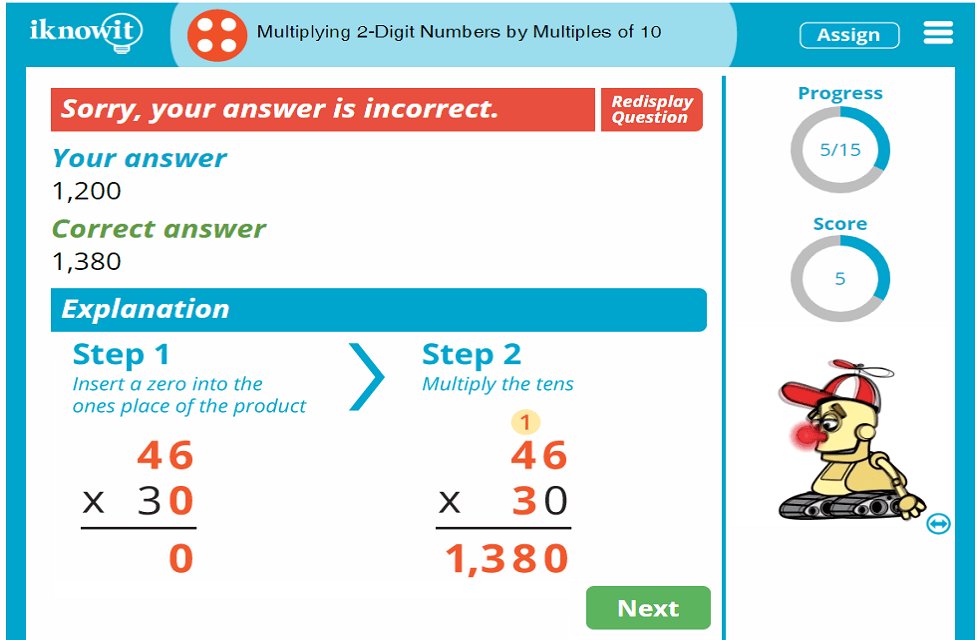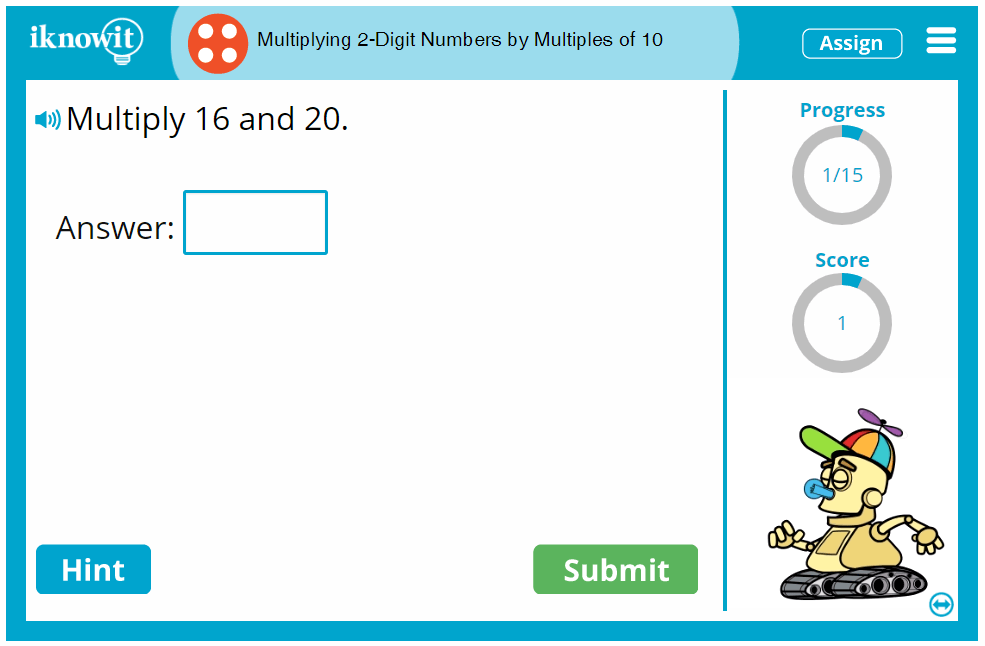## Level

This Level D multiplication lesson may be ideal for your fourth grade class.

## Common Core Standard

4.NBT.5
Number and Operations in Base Ten

Students will use their understanding of place value and the properties of operations to perform multi-digit arithmetic.

## You might also be interested in...

Multiplying 2-Digit by 1-Digit Numbers (Level D)
In this fourth grade-level math lesson, students will practice multiplying 2-digit numbers by 1-digit numbers. This lesson includes vertical multiplication problems, horizontal multiplication problems, and multiplication word problems.

Multiplying 3-Digit by 1-Digit Numbers (Level D)
Students will practice multiplying 3-digit numbers by 1-digit numbers in this interactive math lesson. Math problems include horizontal multiplication problems, vertical multiplication problems, and multiplication word problems.